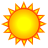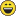# Purpose of the describe object as opposed to native object?

874
2
08-26-2014 12:32 AMNew Contributor II

Hi there

I am trying to determine what benefit the describe object has over using the properties of the original object.

Does the describe object simply have all the properties that were present on the original object, plus some additional useful generic properties that are not present on the original objects (eg feature class, raster, etc).

Also, is there a way to retrieve a full list of an Arc objects properties?

Cheers

Mal

Tags (2)
1 Solution

Accepted SolutionsOccasional Contributor

Hi Mal

I'm assuming your referring to the "Describe Object Properties" aspect of arcpy? e.g

````desc = arcpy.Describe("C:/Data/chesapeake.gdb")`
```

if so the basic Describe functions (As shown here‌) are just some of the basic properties you can access. For Example

````import arcpy# Create a Describe object#desc = arcpy.Describe("C:/Data/chesapeake.gdb") `

`print "DataType: " + desc.dataType`
```

However you can extend this function depending on the type of object your referencing. The example above used a geodatabase workspace. You could for example desibe a feature class. This would give you access to different properties. For Example

````# Create a Describe object from the feature class`
`desc = arcpy.Describe("C:/data/arch.dgn/Point")`

`print "Feature Type: " + desc.featureType`

`print "Shape Type : " + desc.shapeType`

```

Or for a Raster

````desc = arcpy.Describe("C:/data/preston.img")# Print some raster dataset properties# print "Band Count:  %d" % desc.bandCountprint "Compression Type: %s" % desc.compressionTypeprint "Raster Format:  %s" % desc.format`
```

You can get a full list here. I hope that answers your question and isn't just a waffle2 RepliesOccasional Contributor

Hi Mal

I'm assuming your referring to the "Describe Object Properties" aspect of arcpy? e.g

````desc = arcpy.Describe("C:/Data/chesapeake.gdb")`
```

if so the basic Describe functions (As shown here‌) are just some of the basic properties you can access. For Example

````import arcpy# Create a Describe object#desc = arcpy.Describe("C:/Data/chesapeake.gdb") `

`print "DataType: " + desc.dataType`
```

However you can extend this function depending on the type of object your referencing. The example above used a geodatabase workspace. You could for example desibe a feature class. This would give you access to different properties. For Example

````# Create a Describe object from the feature class`
`desc = arcpy.Describe("C:/data/arch.dgn/Point")`

`print "Feature Type: " + desc.featureType`

`print "Shape Type : " + desc.shapeType`

```

Or for a Raster

````desc = arcpy.Describe("C:/data/preston.img")# Print some raster dataset properties# print "Band Count:  %d" % desc.bandCountprint "Compression Type: %s" % desc.compressionTypeprint "Raster Format:  %s" % desc.format`
```

You can get a full list here. I hope that answers your question and isn't just a waffleNew Contributor II

Hi Matthew

Thanks for the response, it does clarify this a little bit.

I think my confusion actually lay in whether object properties could be directly accessed without creating a Describe object. For example, accessing the raster bandCount property from the raster object directly without creating a Describe object.

After a little experimentation I find that this is indeed possible - my problem was that in the method I used of listing datasets then looping through the list, the iterable item was actually unicode and needs to be converted into the correct object first, ie:

```import arcpy
arcpy.env.workspace = r"C:\Users\uqmnunn\Documents\Borneo\spatial_data\DataInventoryTool_test"

datasets = arcpy.ListDatasets("*", "Raster")

for d in datasets:
print("Raster dataset found: %s" % str(d))
print("Band count:%d" % d.bandCount)
```

Will return an AttributeError to say that 'unicode' object has no attribute 'bandCount'. However, converting d to a raster object first makes it possible to access raster object properties directly without a Describe object:

```     d = arcpy.Raster(d)
```

So thank you for the explanation of Describe, and now I have clarified my other misunderstanding about accessing object properties without Describe!

Cheers

Mal